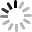# SVG 研究之路 (20) - transform Matrix

## Matrix 的原理

Matrix 就是矩陣，在 SVG 的 transform 裡有六個數值，分別是：matrix(a,b,c,d,e,f)，原本的座標、長寬和角度透過矩陣的運算，就可以轉換出一組新的座標、長寬和角度，而剛剛看到的六個參數，其實真正的長相是下圖這個樣子 ( 圖片來源：www.w3.org )：## Matrix 轉換

• translate：matrix(1 0 0 1 tx ty)

轉換後的 x=x+tx，轉換後的 y=y+ty，也就是我們水平或垂直的位移。

``````  <rect fill="none" width="60" height="50" x="10" y="50" stroke="#000" stroke-width="2" />
<rect fill="#c00" width="60" height="50" x="10" y="50" transform="matrix(1,0,0,1,50,20)" />
``````• scale：matrix(sx 0 0 sy 0 0)

轉換後的 x=sx，轉換後的 y=sy，這裡的 x 和 y 要理解不是座標的 x 和 y，而原本的尺寸大小比例，也就是 1，也因為我們的 transform 是套用在圖形上，所以關於圖形所有的屬性都會依據 Matrix 去做變換，所以不只寬高，連原本左上的座標都會乘上比例而做變化，這也是為什麼我們套用 scale 的時候座標也會跟著變的主要原因。

``````  <rect fill="#0c0" width="60" height="50" x="10" y="10" transform="matrix(2,0,0,2,0,0)" />
<rect fill="none" width="60" height="50" x="10" y="10" stroke="#000" stroke-width="2" />
``````• rotate：matrix(cos(a) sin(a) -sin(a) cos(a) 0 0)

轉換後的 x=cos(a)x-sin(a)y，轉換後的 y=sin(a)x+cos(a)，也因此會預設繞著 (0,0) 的座標去做旋轉，範例程式是順時針旋轉 30 度，sin(30)=0.5，cos(30)=0.866。

``````  <rect fill="#09c" width="60" height="50" x="60" y="10" transform="matrix(0.866,0.5,-0.5,0.866,0,0)" />
<rect fill="none" width="60" height="50" x="60" y="10" stroke="#000" stroke-width="2" />
``````這時候你可能會想問，我們在 rotate(cx,cy) 不是可以設定圓心座標嗎？事實是這樣的，其實 rotate(cx,cy) 事實上是在 matrix 的前後都加上了一個 translate 去做座標的轉換，轉換公式：`rotate(cx,cy) = translate(cx,cy) Matrix(cos(a) sin(a) -sin(a) cos(a) 0 0) translate(-cx,-cy)`藉由這一層的公式轉換，我們就可以將圓心座標重新設定，也讓我們直接寫 rotate(cx,cy) 就好囉！

``````  <rect fill="#09c" width="60" height="50" x="60" y="10" transform="translate(60,10) matrix(0.866,0.5,-0.5,0.866,0,0) translate(-60,-10) " />
<rect fill="none" width="60" height="50" x="60" y="10" stroke="#000" stroke-width="2" />
``````• skewX：matrix(1 0 tan(a) 1 0 0)、skewY：matrix(1 tan(a) 0 1 0 0)

在前一篇我們有提到 skewX 的傾斜變形，之所以會位移是因為 Matrix 裏頭 tan(角度)的原因就在此，其實 skewX 如果會水平位移，是受到 y 的座標影響，而 skewY 會垂直位移，則是受到了 x 座標的影響，這也是在使用 skew 的時候必須要注意的地方。( 傾斜 30 度,tan(30)=0.577 )

從範例程式可以很清楚的看到，因為 y 的座標是 10，所以在經過 skewX 之後，x 的水平位移會增加了 10*0.577 的數值，這也是為什麼會位移的原因。``````  <rect fill="#f80" width="60" height="50" x="10" y="10" transform="matrix(1,0,0.577,1,0,0)" />
<rect fill="none" width="60" height="50" x="10" y="10" stroke="#000" stroke-width="2" />
``````當然我們也可以實驗一下，把 y 的座標設為 0，就不會有水平位移囉！

``````  <rect fill="#f80" width="60" height="50" x="10" y="0" transform="matrix(1,0,0.577,1,0,0)" />
<rect fill="none" width="60" height="50" x="10" y="0" stroke="#000" stroke-width="2" />
``````## 小結

``````<rect fill="#a0a" width="60" height="50" x="10" y="10" transform="matrix(0.866,0.5,-0.5,0.866,0,0) matrix(1,0,0.577,1,0,0) matrix(1,0,0,1,30,0)" />
<rect fill="none" width="60" height="50" x="10" y="10" stroke="#000" stroke-width="2" />
``````